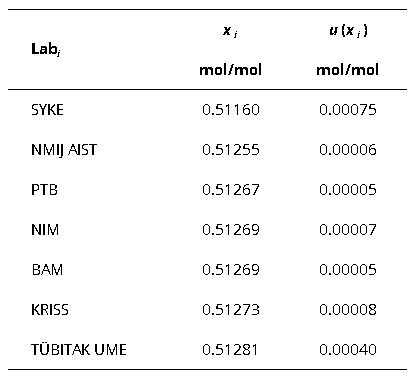Comparison
Comparison close
Results

CCQM-K122

SUBJECT                      Anionic impurities and lead in salt solutions

MEASURAND      Mass fraction of bromide

Degrees of equivalence represented by Di = (xI - xR) and its expanded uncertainty Ui at a 95 % level of confidence, both expressed in µg/g.

Mass fraction of bromide
Di
Participating institutes

CCQM-K122

SUBJECT                      Anionic impurities and lead in salt solutions

MEASURAND      Mass fraction of sulfate

Degrees of equivalence represented by Di = (xI - xR) and its expanded uncertainty Ui at a 95 % level of confidence, both expressed in µg/g.

Mass fraction of sulfate
Di
Participating institutes

CCQM-K122

SUBJECT                      Anionic impurities and lead in salt solutions

Degrees of equivalence represented by Di = (xI - xR) and its expanded uncertainty Ui at a 95 % level of confidence, both expressed in µg/g.

Di
Participating institutes

CCQM-K122

SUBJECT                      Anionic impurities and lead in salt solutions

Degrees of equivalence represented by Di = (xI - xR) and its expanded uncertainty Ui at a 95 % level of confidence, both expressed in g/mol.

Di
Participating institutes

CCQM-K122

SUBJECT                      Anionic impurities and lead in salt solutions

MEASURAND      Amount fraction of Pb-204

Degrees of equivalence represented by Di = (xI - xR) and its expanded uncertainty Ui at a 95 % level of confidence, both expressed in mol/mol.

Amount fraction of Pb-204
Di
Participating institutes

CCQM-K122

SUBJECT                      Anionic impurities and lead in salt solutions

MEASURAND      Amount fraction of Pb-206

Degrees of equivalence represented by Di = (xI - xR) and its expanded uncertainty Ui at a 95 % level of confidence, both expressed in mol/mol.

Amount fraction of Pb-206
Di
Participating institutes

CCQM-K122

SUBJECT                      Anionic impurities and lead in salt solutions

MEASURAND      Amount fraction of Pb-207

Degrees of equivalence represented by Di = (xI - xR) and its expanded uncertainty Ui at a 95 % level of confidence, both expressed in mol/mol.

Amount fraction of Pb-207
Di
Participating institutes

CCQM-K122

SUBJECT                      Anionic impurities and lead in salt solutions

MEASURAND      Amount fraction of Pb-208

Degrees of equivalence represented by Di = (xI - xR) and its expanded uncertainty Ui at a 95 % level of confidence, both expressed in mol/mol.

Amount fraction of 208Pb
Di
Participating institutes
Comparison
Comparison close
Results

CCQM-K122

SUBJECT                      Anionic impurities and lead in salt solutions

MEASURAND      Mass fraction of bromide

Degrees of equivalence represented by Di = (xI - xR) and its expanded uncertainty Ui at a 95 % level of confidence, both expressed in µg/g.

LABi Di Uneg,i Upos,i
µg/g
µg/g
µg/g
KRISS -1.03 0.52
INTI -1.01 0.32
TÜBITAK UME -0.33 0.35
SMU -0.21 0.77
NIMT -0.21 0.28
SMU -0.19 0.84
PTB 0.00 0.31
NIM 0.04 0.29
NRC 0.15 0.26
GUM 0.15 0.33
NMIJ AIST 0.20 0.34
CENAM 0.85 1.42
Participating institutes
CCQM-K122

CCQM-K122

SUBJECT                      Anionic impurities and lead in salt solutions

MEASURAND      Mass fraction of sulfate

Degrees of equivalence represented by Di = (xI - xR) and its expanded uncertainty Ui at a 95 % level of confidence, both expressed in µg/g.

LABi Di Uneg,i Upos,i
µg/g
µg/g
µg/g
CENAM -0.45 0.67
KRISS -0.31 0.32
PTB -0.07 0.31
INTI -0.04 0.37
NMIJ AIST 0.00 0.30
NIM 0.21 0.31
GUM 0.21 0.34
SMU 0.23 0.33
TÜBITAK UME 0.45 0.43
Participating institutes
CCQM-K122

CCQM-K122

SUBJECT                      Anionic impurities and lead in salt solutions

Degrees of equivalence represented by Di = (xI - xR) and its expanded uncertainty Ui at a 95 % level of confidence, both expressed in µg/g.

LABi Di Uneg,i Upos,i
µg/g
µg/g
µg/g
SYKE -0.0010 0.0007
TÜBITAK UME -0.0002 0.0009
NRC -0.0002 0.0006
NIM -0.0001 0.0006
NMIJ AIST 0.0001 0.0003
BAM 0.0001 0.0003
PTB 0.0002 0.0008
KRISS 0.0005 0.0008
Participating institutes
CCQM-K122

CCQM-K122

SUBJECT                      Anionic impurities and lead in salt solutions

Degrees of equivalence represented by Di = (xI - xR) and its expanded uncertainty Ui at a 95 % level of confidence, both expressed in g/mol.

LABi Di Uneg,i Upos,i
g/mol
g/mol
g/mol
NMIJ AIST -0.00050 0.00053
PTB -0.00021 0.00031
BAM -0.00016 0.00031
NIM 0.00000 0.00033
KRISS 0.00001 0.00072
SYKE 0.00010 0.00190
TÜBITAK UME 0.00020 0.00133
Participating institutes
CCQM-K122

CCQM-K122

SUBJECT                      Anionic impurities and lead in salt solutions

MEASURAND      Amount fraction of Pb-204

Degrees of equivalence represented by Di = (xI - xR) and its expanded uncertainty Ui at a 95 % level of confidence, both expressed in mol/mol.

LABi Di Uneg,i Upos,i
mol/mol
mol/mol
mol/mol
TÜBITAK UME -0.00004 0.00005
NMIJ AIST -0.00001 0.00001
BAM 0.00000 0.00001
PTB 0.00000 0.00001
KRISS 0.00000 0.00001
NIM 0.00001 0.00019
SYKE 0.00013 0.00016
Participating institutes
CCQM-K122

CCQM-K122

SUBJECT                      Anionic impurities and lead in salt solutions

MEASURAND      Amount fraction of Pb-206

Degrees of equivalence represented by Di = (xI - xR) and its expanded uncertainty Ui at a 95 % level of confidence, both expressed in mol/mol.

LABi Di Uneg,i Upos,i
mol/mol
mol/mol
mol/mol
NIM -0.0001 0.0002
KRISS -0.0001 0.0003
TÜBITAK UME -0.0001 0.0005
BAM 0.0000 0.0002
PTB 0.0000 0.0002
NMIJ AIST 0.0002 0.0003
SYKE 0.0006 0.0013
Participating institutes
CCQM-K122

CCQM-K122

SUBJECT                      Anionic impurities and lead in salt solutions

MEASURAND      Amount fraction of Pb-207

Degrees of equivalence represented by Di = (xI - xR) and its expanded uncertainty Ui at a 95 % level of confidence, both expressed in (unit).

LABi Di Uneg,i Upos,i
mol/mol
mol/mol
mol/mol
NMIJ AIST -0.00006 0.00012
TÜBITAK UME -0.00001 0.00054
BAM 0.00000 0.00013
PTB 0.00000 0.00013
KRISS 0.00009 0.00014
NIM 0.00012 0.00016
SYKE 0.00029 0.00101
Participating institutes
CCQM-K122

CCQM-K122

SUBJECT                      Anionic impurities and lead in salt solutions

MEASURAND      Amount fraction of Pb-208

Degrees of equivalence represented by Di = (xI - xR) and its expanded uncertainty Ui at a 95 % level of confidence, both expressed in mol/mol.

LABi Di Uneg,i Upos,i
mol/mol
mol/mol
mol/mol
SYKE -0.00109 0.00150
NMIJ AIST -0.00014 0.00014
PTB -0.00002 0.00012
NIM 0.00000 0.00015
BAM 0.00000 0.00012
KRISS 0.00004 0.00021
TÜBITAK UME 0.00012 0.00079
Participating institutes
CCQM-K122
Comparison
Comparison close
CC comparison
 Metrology area, Sub-field Chemistry and Biology, Inorganics Description Anionic impurities (Bromide, Sulfate) and Lead in salt solutions Time of measurements 2014 Status Approved for equivalence
 Final Reports of the comparisons Measurand Mass fraction of Bromide, Sulfate and Lead in the sample Transfer device Dedicated samples
 Comparison type Key Comparison Consultative Committee CCQM (Consultative Committee for Amount of Substance) Conducted by CCQM (Consultative Committee for Amount of Substance) Pilot institute PTB Physikalisch-Technische Bundesanstalt Germany Contact person Olaf Rienitz olaf.rienitz@ptb.de +49 531 592 3110
First Name Last Name
wwww@ww.www +356719836 Institute 1 Institute 1 Khmelnitskiy
Pilot laboratory
PTB

Physikalisch-Technische Bundesanstalt, Germany, EURAMET

 BAM Bundesanstalt für Materialforschung und -prüfung, Germany, EURAMET
 CENAM Centro Nacional de Metrologia, Mexico, SIM
 GUM Glowny Urzad Miar, Central Office of Measures, Poland, EURAMET
 INMETRO Instituto Nacional de Metrologia, Qualidade e Tecnologia, Brazil, SIM
 INTI Instituto Nacional de Tecnologia Industrial, Argentina, SIM
 KRISS Korea Research Institute of Standards and Science, Korea, Republic of, APMP
 MIKES-SYKE Finnish Environment Institute, Finland, EURAMET
 NIM National Institute of Metrology, China, APMP
 NIMT National Institute of Metrology (Thailand), Thailand, APMP
 NMIJ AIST National Metrology Institute of Japan, Japan, APMP
 NRC National Research Council, Canada, SIM
 SMU Slovensky Metrologicky Ustav, Slovakia, EURAMET
 UME TÜBITAK Ulusal Metroloji Enstitüsü, Turkey, EURAMET
Comparison
Comparison close
Results

CCQM-K122

SUBJECT                      Anionic impurities and lead in salt solutions

MEASURAND      Mass fraction of bromide

The key comparison reference value xR and its associated standard uncertainty uR are determined from the median.

The degree of equivalence of laboratory i relative to the key comparison reference value xR is given by a pair of terms, both expressed in the same unit:

Di = (xi - xR) and its expanded uncertainty Ui at a 95 % level of confidence.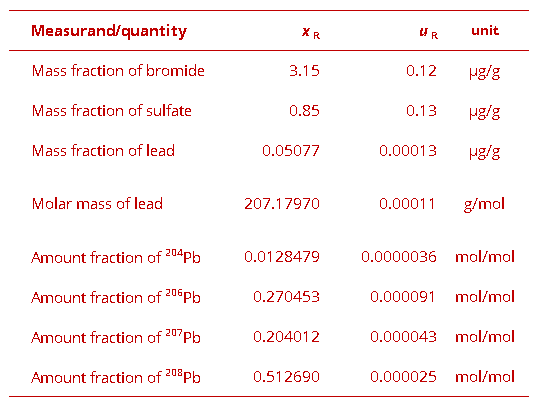CCQM-K122

SUBJECT                      Anionic impurities and lead in salt solutions

MEASURAND      Mass fraction of sulfate

The key comparison reference value xR and its associated standard uncertainty uR are determined from the median.

The degree of equivalence of laboratory i relative to the key comparison reference value xR is given by a pair of terms, both expressed in the same unit:

Di = (xi - xR) and its expanded uncertainty Ui at a 95 % level of confidence.CCQM-K122

SUBJECT                      Anionic impurities and lead in salt solutions

The key comparison reference value xR and its associated standard uncertainty uR are determined from the median.

The degree of equivalence of laboratory i relative to the key comparison reference value xR is given by a pair of terms, both expressed in the same unit:

Di = (xi - xR) and its expanded uncertainty Ui at a 95 % level of confidence.CCQM-K122

SUBJECT                      Anionic impurities and lead in salt solutions

The key comparison reference value xR and its associated standard uncertainty uR are determined from the median.

The degree of equivalence of laboratory i relative to the key comparison reference value xR is given by a pair of terms, both expressed in the same unit:

Di = (xi - xR) and its expanded uncertainty Ui at a 95 % level of confidence.CCQM-K122

SUBJECT                      Anionic impurities and lead in salt solutions

MEASURAND      Amount fraction of Pb-204

The key comparison reference value xR and its associated standard uncertainty uR are determined from the median.

The degree of equivalence of laboratory i relative to the key comparison reference value xR is given by a pair of terms, both expressed in the same unit:

Di = (xi - xR) and its expanded uncertainty Ui at a 95 % level of confidence.CCQM-K122

SUBJECT                      Anionic impurities and lead in salt solutions

MEASURAND      Amount fraction of Pb-206

The key comparison reference value xR and its associated standard uncertainty uR are determined from the median.

The degree of equivalence of laboratory i relative to the key comparison reference value xR is given by a pair of terms, both expressed in the same unit:

Di = (xi - xR) and its expanded uncertainty Ui at a 95 % level of confidence.CCQM-K122

SUBJECT                      Anionic impurities and lead in salt solutions

MEASURAND      Amount fraction of Pb-207

The key comparison reference value xR and its associated standard uncertainty uR are determined from the median.

The degree of equivalence of laboratory i relative to the key comparison reference value xR is given by a pair of terms, both expressed in the same unit:

Di = (xi - xR) and its expanded uncertainty Ui at a 95 % level of confidence.CCQM-K122

SUBJECT                      Anionic impurities and lead in salt solutions

MEASURAND      Amount fraction of Pb-208

The key comparison reference value xR and its associated standard uncertainty uR are determined from the median.

The degree of equivalence of laboratory i relative to the key comparison reference value xR is given by a pair of terms, both expressed in the same unit:

Di = (xi - xR) and its expanded uncertainty Ui at a 95 % level of confidence.Comparison
Comparison close
Results

CCQM-K122

SUBJECT                      Anionic impurities and lead in salt solutions

MEASURAND      Mass fraction of bromide

xi   measurement value reported by laboratory iLabi

ui   combined standard uncertainty of xi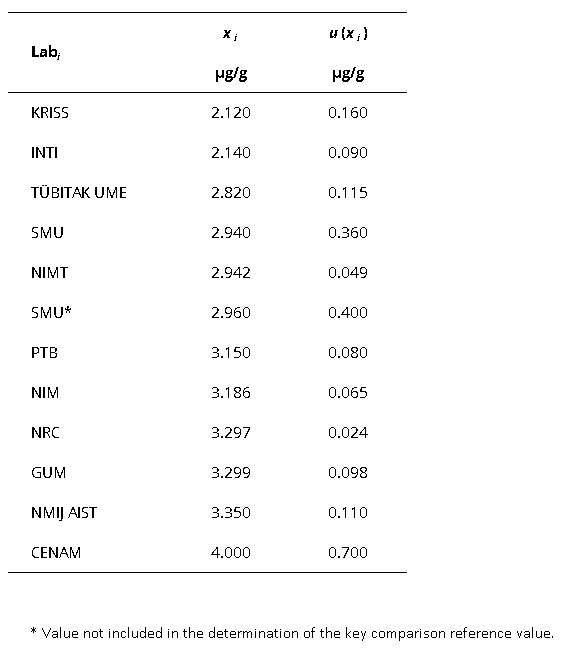CCQM-K122

SUBJECT                      Anionic impurities and lead in salt solutions

MEASURAND      Mass fraction of sulfate

xi   measurement value reported by laboratory iLabi

ui   combined standard uncertainty of xi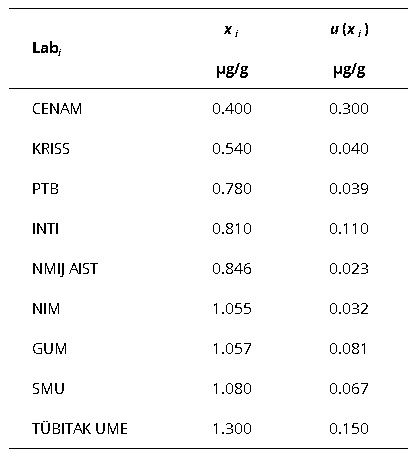CCQM-K122

SUBJECT                      Anionic impurities and lead in salt solutions

xi   measurement value reported by laboratory iLabi

ui   combined standard uncertainty of xi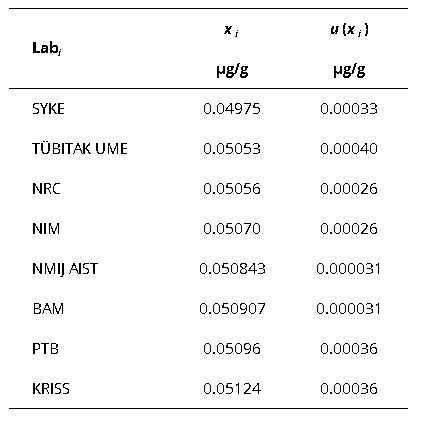CCQM-K122

SUBJECT                      Anionic impurities and lead in salt solutions

xi   measurement value reported by laboratory iLabi

ui   combined standard uncertainty of xi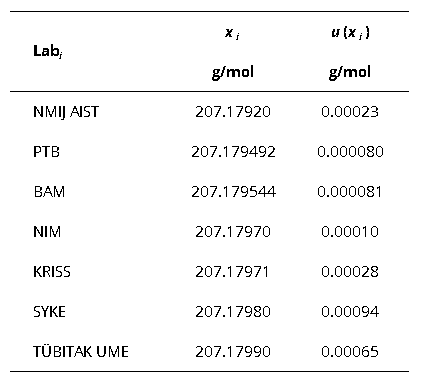CCQM-K122

SUBJECT                      Anionic impurities and lead in salt solutions

MEASURAND      Amount fraction of Pb-204

xi   measurement value reported by laboratory iLabi

ui   combined standard uncertainty of xi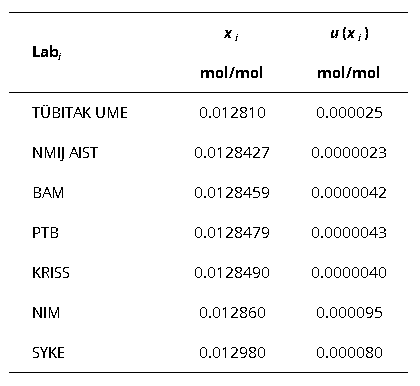CCQM-K122

SUBJECT                      Anionic impurities and lead in salt solutions

MEASURAND      Amount fraction of Pb-206

xi   measurement value reported by laboratory iLabi

ui   combined standard uncertainty of xi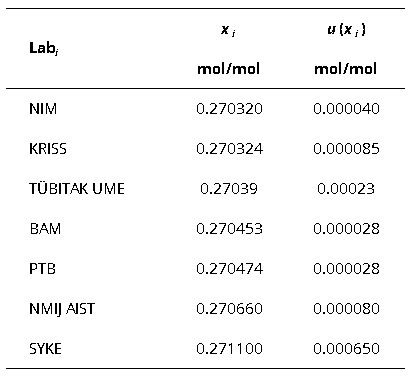CCQM-K122

SUBJECT                      Anionic impurities and lead in salt solutions

MEASURAND      Amount fraction of Pb-207

xi   measurement value reported by laboratory iLabi

ui   combined standard uncertainty of xi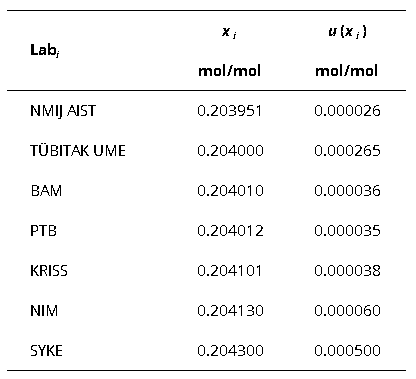CCQM-K122

SUBJECT                      Anionic impurities and lead in salt solutions

MEASURAND      Amount fraction of Pb-208

xi   measurement value reported by laboratory iLabi

ui   combined standard uncertainty of xi